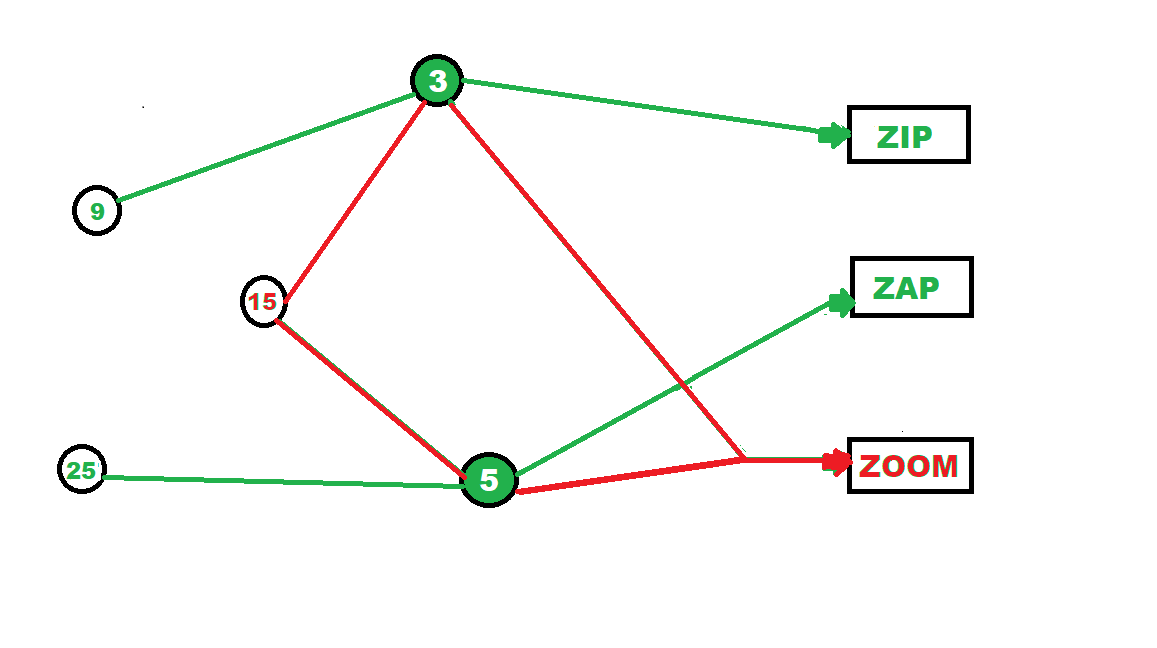Open in App
Not now

# Python program for Zip, Zap and Zoom game

• Last Updated : 30 Nov, 2022

Zip zap zoom is a fun game that requires its players to concentrate and participate actively. When this game is played with people, this is how it is played:

• Players stand in a circle, 6 feet away from each other
• One player starts the game by clap-pointing and saying zap to the person on its left
• This person now does the same to the person to its right and says zap
• Third person so selected now can choose anyone irrespective of the direction by saying zoom
• Now the person so selected starts the game again with zap and the continues in this fashion
• If somebody messes up in between the game is restarted.

In Python programming, this game will be achieved by the following approach.

### Approach:

• Take in a number as input from the user.
• Check if the number is a multiple of both 3 and 5, if it is then print “Zoom”.
• If it is not, then check if the number is a multiple of 3, if it is then print “Zip”.
• If it is a multiple of 5 instead, print “Zap”.
• Else, print a default statement.

Example:

```Input:6
Output:
Zip```

#### Here is a simple and fun illustration describing the same:Program:

## C++

 `#include ``using` `namespace` `std;` `void` `zzz(``int` `number)``{``    ``if` `(number % 3 == 0 && number % 5 == 0)``        ``cout << ``"Zoom\n"``;``    ``else` `if` `(number % 3 == 0)``        ``cout << ``"Zip\n"``;``    ``else` `if` `(number % 5 == 0)``        ``cout << ``"Zap\n"``;``    ``else``        ``cout << ``"Invalid number!\n"``;``}` `// Driver code``int` `main()``{` `    ``// Calling function``    ``zzz(7);``    ``zzz(6);``    ``zzz(5);``    ``zzz(30);` `    ``return` `0;``}` `// This code is contributed by svrrrsvr`

## Python3

 `def` `main():``    ``zzz(``7``)``    ``zzz(``6``)``    ``zzz(``5``)``    ``zzz(``30``)`  `def` `zzz(number):``    ``if` `number ``%` `3` `=``=` `0` `and` `number ``%` `5` `=``=` `0``:``        ``print``(``"Zoom"``)``    ``elif` `number ``%` `3` `=``=` `0``:``        ``print``(``"Zip"``)``    ``elif` `number ``%` `5` `=``=` `0``:``        ``print``(``"Zap"``)``    ``else``:``        ``print``(``"Invalid number!"``)`  `main()`

Output:

```Invalid number!
Zip
Zap
Zoom```

Code Explanation:

1. The code in main() calls the zzz() function seven times.
2. The first time, it passes in 7 as the argument.
3. The second time, it passes in 6.
4. The third time, it passes in 5.
5. The fourth time, it passes in 30.
6. The zzz() function takes an integer argument and returns a string of text depending on whether that number is divisible by 3 or 5 (or both).
7. If the number is divisible by 3, the function prints “Zoom.”
8. If the number is divisible by 5, the function prints “Zip.”
9. Otherwise, the function prints “Invalid number!”
10. In order to understand what’s going on inside of zzz(), you need to know about basic programming concepts like variables and functions.
11. A variable is a name given to a piece of data that can be used within a program.
12. Functions are special pieces of code that perform specific tasks (like printing out text).
13. When you call a function from another part of your program (like main()), your computer will automatically start executing that particular function’s code right away.
14. The code will output the following: Zoom Zip Zap.

My Personal Notes arrow_drop_up